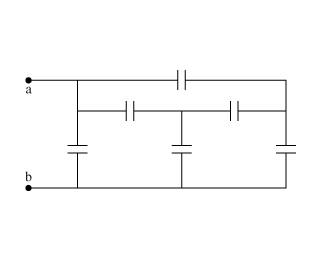# Equivalent capacitance in complex circuit

## Homework Statement

Hello, I was given this problem as a homework assigment.
Each capacitor in the figure has capacitance C. What is the equivalent capacitance between points a and b?
The answer is given in "C_eq/C"## Homework Equations

I used the "C_eq={(1/C_1)+(1/C_2)+.....(1/C_n)}^-1" for the capacitors in series.
I used the "C_eq=(C_1)+(C_2)+.....(C_n) for the capacitors in parallel.

## The Attempt at a Solution

Combining everything that was in parallel and everything that was in series until it come down to one capacitor, the answer that i get is C or 1. Any ideas on what I am doing wrong or if im inputing the answer in the wrong way?

Last edited:

Redbelly98
Staff Emeritus